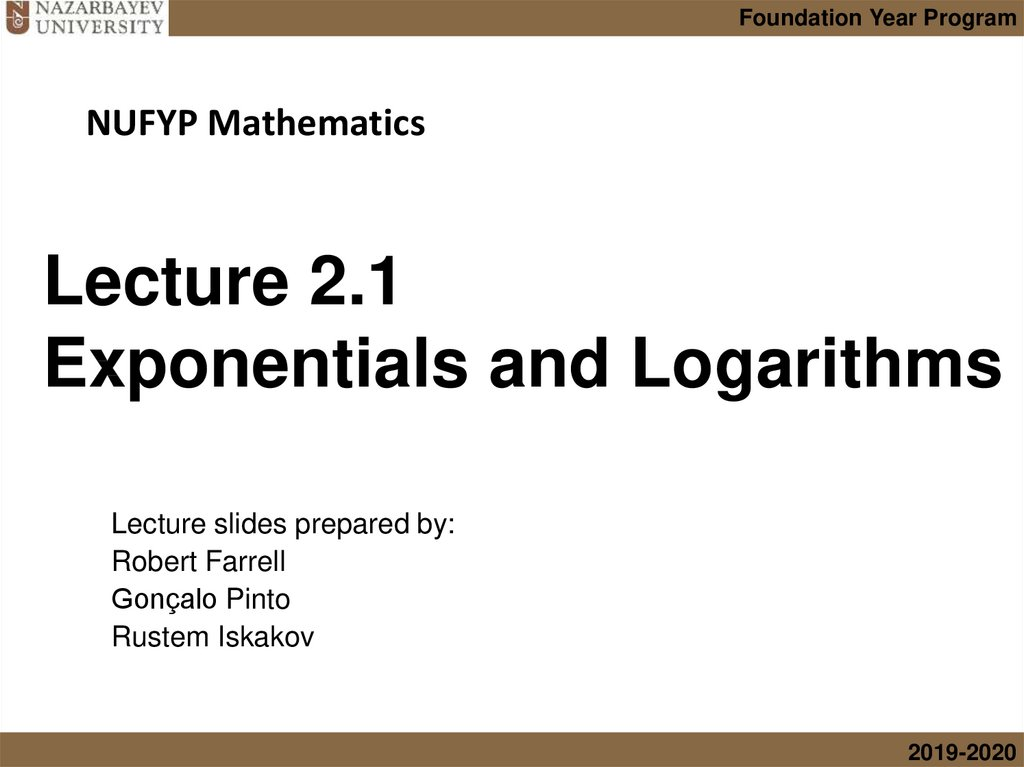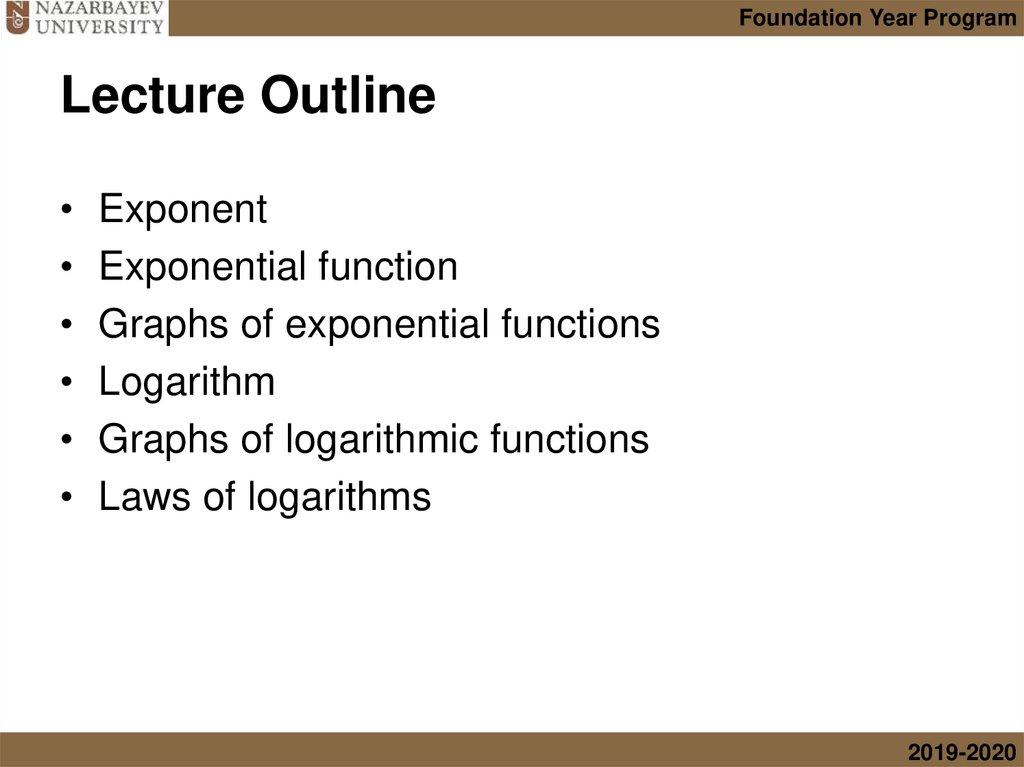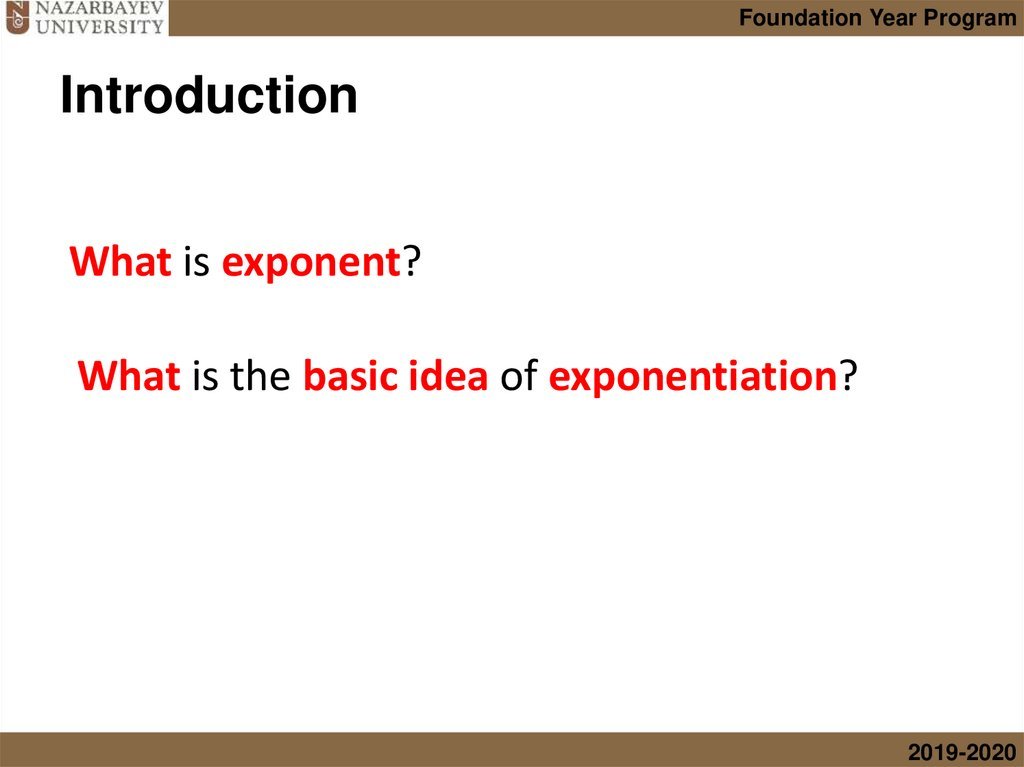# Exponentials and logarithms. Lecture 2.1

## 1. Lecture 2.1 Exponentials and Logarithms

Foundation Year Program
NUFYP Mathematics
Lecture 2.1
Exponentials and Logarithms
Lecture slides prepared by:
Robert Farrell
Gonçalo Pinto
Rustem Iskakov
2019-2020

## 2. Lecture Outline

Foundation Year Program
Lecture Outline
Exponent
Exponential function
Graphs of exponential functions
Logarithm
Graphs of logarithmic functions
Laws of logarithms
2019-2020

## 3. Introduction

Foundation Year Program
Introduction
What is exponent?
What is the basic idea of exponentiation?
2019-2020

## 4. Introduction

Foundation Year Program
Introduction
What is exponent? Exponent is an index or power.
What is the basic idea of exponentiation?
2019-2020

## 5. Introduction

Foundation Year Program
Introduction
What is the basic idea of exponentiation?
5
2019-2020

## 6. Introduction

Foundation Year Program
Introduction
What is the basic idea of exponentiation?
Repeated
Repeated
multiplication
6
2019-2020

## 7.

Foundation Year Program
Exponential function
An exponential function has the form
Exponent, index, power
(variable)
f(x) = ax
• where a is constant
• a ≠1, a > 0
Examples:
base
f (x) = 2x , f(x) = 3x , f(x) = ex
7
2019-2020

## 8.

Foundation Year Program
2.1.1 Sketch the graph of Exponential function
Let us see some graphs of exponential functions with
different bases on the same axes:
The larger the base ⇾ steeper the graph
y-intercept (0; 1)
8
2019-2020

## 9.

Foundation Year Program
An exponential function has the form
Exponent, index, power
(variable)
Why a ≠1?
f(x) = ax
• where a is constant
• a ≠1, a > 0
base
Examples: